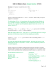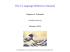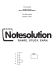# 15-122 : Principles of Imperative Computation, Spring 2013 Homework 2 Theory

## Transcription

15-122 : Principles of Imperative Computation, Spring 2013 Homework 2 Theory
```15-122 Homework 2
Page 1 of 8
15-122 : Principles of Imperative Computation, Spring 2013
Homework 2 Theory
Due: Tuesday, February 12, 2013, at the beginning of lecture
Name:
Andrew ID:
Recitation:
The written portion of this week’s homework will give you some practice working with
searching algorithms and test your understanding of contracts.
Question Points Score
1
6
2
4
3
10
Total:
20
You must use this printout, include this cover sheet,
and staple the whole thing together before turning it in.
Either type up the assignment using 15122-theory2.tex,
15-122 Homework 2
Page 2 of 8
1. Reasoning with Invariants. Consider the following implementation of the linear
search algorithm that finds the first occurrence of x in array A:
/*
/*
/*
/*
/*
/*
/*
/*
/*
/*
/*
/*
/*
/*
/*
1
2
3
4
5
6
7
8
9
10
11
12
13
14
15
*/
*/
*/
*/
*/
*/
*/
*/
*/
*/
*/
*/
*/
*/
*/
int find_first(int x, int[] A, int n)
//@requires 0 <= n && n <= \length(A);
//@requires is_sorted(A, 0, n);
//@ensures (\result == -1 && !is_in(x, A, 0, n))
|| (A[\result] == x && !is_in(x, A, 0, \result));
{
int i = 0;
while (i < n && A[i] <= x)
{
if (A[i] == x) return i;
i = i + 1;
}
return -1;
}
The function is sorted has the following signature:
bool is_sorted(int[] A, int lower, int upper)
//@requires 0 <= lower && lower <= \length(A) - 1;
//@requires 0 <= upper && upper <= \length(A);
//@requires lower <= upper;
;
and returns true if the array A is sorted in increasing order from [lower, upper).
You may also use the function is in in any of the following questions. Its signature is:
bool is_in(int x, int[] A, int lower, int upper)
//@requires 0 <= lower && lower <= upper && upper <= \length(A);
;
and it returns true if A[i] == x for some i in [lower, upper).
15-122 Homework 2
(2)
Page 3 of 8
(a) Add loop invariants to the while loop in the code and show that they hold for this
loop. Be sure that the loop invariants precisely describe the computation in the
loop and that they imply safety of the array access in the while loop.
Solution:
Loop invariant(s):
The loop invariant(s) hold(s) initially:
The loop invariant(s) is/are preserved by every iteration of the loop:
15-122 Homework 2
(4)
Page 4 of 8
(b) Show that the loop invariant is strong enough by using the loop invariant to prove
that the postconditions hold when the function returns. You do not need to prove
the loop invariant’s correctness here (you already did that in 1.a), but you might
need to change that answer to part 4(a) in order for the loop invariant to actually
imply the function’s correctness. Make sure to deal with the fact that find can
return in two different ways.
Solution:
If the function returns on line 11:
If the function returns on line 14:
15-122 Homework 2
Page 5 of 8
2. Runtime Complexity. Consider the following function that sorts the integers in an
array.
void sort(int[] A, int n)
//@requires 0 <= n && n <= \length(A);
{
int i = 1;
while (i < n)
//@loop_invariant 0 <= i;
{
int j = i;
while (j != 0 && A[j-1] > A[j])
//@loop_invariant 0 <= j && j <= i;
{
swap(A, j-1, j);
// function that swaps A[j-1] with A[j]
j = j - 1;
}
i = i + 1;
}
}
(1)
(a) Let T (n) be the worst-case number of swaps made when sort(A, n) is called. Find
an exact expression for T (n).
Solution:
15-122 Homework 2
(1)
Page 6 of 8
(b) Using big-O notation, what is asymptotic complexity of T (n)? This is the worstcase runtime complexity of sort.
Solution:
T (n) = O(
(2)
(c) Using your answer from the previous part, prove that T (n) = O(f (n)) using the
formal definition of big O. That is, find c > 0 and n0 ≥ 0 such that for every
n ≥ n0 , T (n) ≤ cf (n).
Solution:
15-122 Homework 2
Page 7 of 8
3. Computing Overlaps
In this problem, we will study the Overlap Problem, which is the task of computing
the number of shared elements between two arrays. The inputs to this problem will
be an array A[] of m integers and a second array B[] of k integers. We require the
integers of A[] and B[] to be distinct, meaning no integer will occur more than once
in A[] (or in B[]), though some integers may occur once in each of A[] and B[].
Consider the following piece of pseudocode which counts the number of integers
which are in both of A[] and B[].
OverlapCounter(A[], m, B[], k)
• Initialize an integer count to 0.
• For each integer 0 ≤ i < m:
– Use a linear search algorithm to determine if the integer A[i] can be found
in B[]. If so, increment count.
• Output count.
(2)
(a) What is the big-O runtime of this algorithm? Your answer should be in terms of m
and k.
Solution:
(3)
(b) Oftentimes, a problem involving arrays can be solved faster if at least one of the
arrays is assumed to be sorted. In this part, we will assume that that the input array
B[] is sorted, while A[] remains unsorted. Using this assumption, explain how to
modify OverlapCounter to solve the Overlap Problem asymptotically faster than
it currently does.
Solution:
15-122 Homework 2
(2)
Page 8 of 8
m and k.
Solution:
(3)
(d) Sorting arrays is one of the most well-studied problems in all of Computer Science,
and there are several extremely fast algorithms which solve it. Assuming you have
access to a function which sorts an n-element array in time O(n log n), explain
how to solve the Overlap Problem for two (possibly unsorted) arrays A[] and B[]
asymptotically faster than the original version of OverlapCounter. What is the
to devise the fastest algorithm possible.
Solution:
```

### How to install RCS/RK TAGHeuer By Chronelec active loop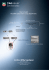### TEMPLATE HOW-TO MAKE A GUM PASTE BOW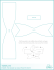### Lab 7 - ocal.info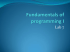### ICSE Computer Application Sample Papers SECTION A (40 Marks)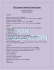### Overview of the Bike Course (radar and Smedleys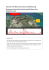### Sample Midterm CSE 680: Introduction to Algorithms and Data Structures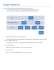### peaked mountain (333 acres) - The Trustees of Reservations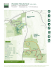### Clivet P-Matic for WLHP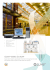### Welcome to the Fitness Trails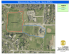### RMFL - Blue Force Gear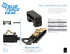### Kokopelli Loops### P P P P P P P P P P P P### 15–122: Principles of Imperative Computation M E 1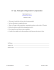### Kokopelli Loops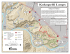### CSC 171 Sample Quiz 6 C Language Programming for Engineers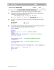### CS 211: Sorting with Merges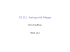### Document 6537043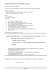### a basis for the constructive approach to programming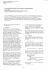### CSc 345: Homework 3 Solution (Written Part)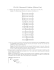### (a) Why is the program made from a.c and b.c... happen if it is executed? CSE 333 Midterm Exam